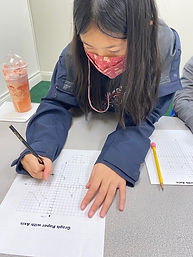## Ms. Dawn

### Target 1​

Continuation

Number Operation

:

Computation

###### 1:

Understand the arithmetic process used for adding and subtracting fractions with unlike denominators.

###### 2:

Recognize that the first step in adding or subtracting fractions with unlike denominators is to create equivalent fractions that share the same denominator.

###### 3:

Simplify the results of an addition or subtraction problem.

4th

###### Vocabulary:

Common denominator, Fraction, Improper fraction, Unlike denominators

Activities:

1. Students completed a few addition and subtraction of fraction problems with unlike denominators.

2. After a few review problems, students played a game called, "Bump." Students took turns rolling two dice. They needed to add both dice up. Then, each sum had a addition fraction problem with unlike denominators. Students needed to solve it correctly in order to secure the spot with the answer. Students could lock it if they were able to place two colored pieces on that answer. They could also bump the other player off if that player only had one of their colored pieces on the spot. The idea of the game is to lock as many as you can. The game eneded when all the spaces were locked.### Home Exploration

###### Guiding Questions:## Absent Students:

### Target 2

:

###### 1:

Multiply a fraction and a whole number using visual models.

###### 2:

Understand how the concept of multiplication can be applied to fractions.

4th

###### Vocabulary:

Multiply, Fraction, Whole number, CUBES, Equations, Number sentences

Activities:

1. Students used prior strategies (CUBES) learned to solve word problems invovling the multiplicaiton of a whole number and a fraction.

2. Students drew pictures (visuals) to help them solve the word problem and to show their thinking.

3. They also wrote number sentences (equations).### Home Exploration

###### Guiding Questions:### Target 3

:

###### 1:

Understand that geometric figures can be drawn on the coordinate plane.

###### 2:

Draw quadrilaterals on the coordinate plane.

4th

###### Vocabulary:

Coordinates, X-axis, Y-axis, Geometric figures, Coordinate plane, Quadrilaterals, Positive, Negative,

Activities:

1. Had a discussion with students about what defines a quadrilateral. After defining what a quadrilateral was, students discussed the x-axis and y-axis.

2. Discussed the rules of x-axis always being the first cooridate in the pair and the y-axis being the second number.

3. Students drew three different quadrilaterals on the coordinate plane. After drawing the three quadrilaterals, they labeld the points for each one. After labeling it, students wrote the point and what the coordinates for that point was. Then, they read it outloud.### Home Exploration